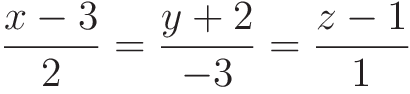Select Page

# Vector Algebra 12 Science Maths CBSE Answers for MCQ in English

Vector Algebra 12 Science Maths CBSE Answers for MCQ in English to enable students to get Answers in a narrative video format for the specific question.

Expert Teacher provides Vector Algebra 12 Science Maths CBSE Answers for MCQ through Video Answers in English language. This video solution will be useful for students to understand how to write an answer in exam in order to score more marks. This teacher uses a narrative style for a question from Vector Algebra not only to explain the proper method of answering question, but deriving right answer too.

Please find the question below and view the Answer in a narrative video format.

Question:

## Similar Questions from CBSE, 12th Science, Maths, Vector Algebra

Question 1 : Find a unit vector parallel to the sum of vectorsand(View Answer Video)

Question 2 : Write a unit vector in the direction of the sum of vectors :and(View Answer Video)

Question 3 : Find the direction cosines of the vector joining the points A(1, 2, -3) and B(-1, -2, 1), directed from A to B. (View Answer Video)

Question 4 :  P and Q are two points with position vectorsandrespectively. Write the position vector of a point R which divides the line segment PQ externally in the ratio 2:1.  (View Answer Video)

Question 5 : For given vectors,andfind the unit vector in the direction of the vector a + b. (View Answer Video)

### Three Dimensional Geometry

Question 1 : Find the distance of the pointfrom the plane 3x + y - z + 2+0 measured parallel to the lineAlso, find the foot of the perpendicular from the given point upon the given plane. (View Answer Video)

Question 2 : Find the vector equation of the line passing through the point (1, 2, 3) and parallel to the planesand(View Answer Video)

Question 3 : Find the vector and cartesian equations of the plane passing through the point (-1, 3, 2) and perpendicular to each of the planes x + 2y +3z = 5 and

Question 4 : Find the equation of the plane passing through the line of intersection of the planesandwhich is perpendicular to the plane. (View Answer Video)

Question 5 : Find the vector equation of a plane which is at a distance of 5 units from the origin and its normal vector is(View Answer Video)

### Inverse Trigonometric Functions

Question 1 : Ifthen find the value of x. (View Answer Video)

Question 2 :is equal to: (View Answer Video)

Question 3 : Solve for(View Answer Video)

Question 4 : Write the value of(View Answer Video)

Question 5 : Ifthen write the value of x + y + xy. (View Answer Video)

### Application of Derivatives

Question 1 : Find the approximate value of f(5.001) where. (View Answer Video)

Question 2 : The point on the curvewhich is nearest to the point (0, 5) is :
Question 4 : Find the equation of the normal to a curvewhich passes through the point (1,2). (View Answer Video)
Question 5 : The normal at the point (1, 1) on the curveis; (View Answer Video)